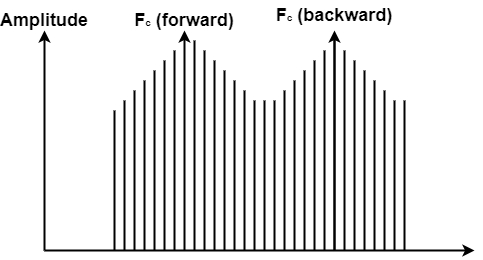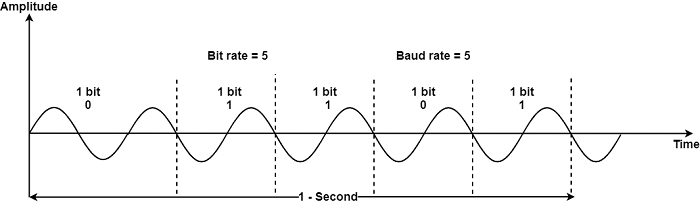# Explain Frequency Shift Keying (FSK) in Computer Network

Computer NetworkNetworkOperating System

#### Artificial Neural Network and Machine Learning using MATLAB

54 Lectures 4 hours

In frequency-shift keying (FSK), the carrier signal frequency is varied to illustrate binary 1 or 0. The frequency of the signal during each bit period is constant, and its value based on the bit (0 or 1) both peak amplitude and phase remain constant.

The figure provides the conceptual view of FSK −FSK prevents most of the noise issues of ASK. Because the receiving device considers definite frequency changes over a given number of time, it can neglect voltage spikes. The limiting aspects of FSK are the physical efficiency of the carrier.## Bandwidth for FSK

Although FSK changes between two carrier frequencies, it is simpler to analyze two coexisting frequencies. The FSK spectrum is the consolidation of two ASK spectra centred around The bandwidth needed for FSK transmission is similar to the band rate of the signal plus the frequency change (the difference between the two carrier frequencies) − $BW=(f_{c_{1}}-f_{c_{0}})+N_{band}$ .

Although these are only two carrier frequencies, the modulation phase generates a composite signal combined with many easy signals, each with multiple frequencies.

Relationship between band rate and bandwidth is $FSK\:BW=(f_{c_{1}}-f_{c_{0}})+N_{band}$

Example − Find the minimum bandwidth for an FSK signal transmitting at 1000 bps. Transmission is in half-duplex mode, and the carriers must be divided by 2000 Hz.

Solution

For FSK, if are the carrier frequencies, then.

$BW=Band rate+(f_{c_{1}}-f_{c_{0}})a$

However, the band rate here is the same as the bit rate. Therefore

$BW=Band rate+(f_{c_{1}}-f_{c_{0}})$

=1000+2000=3000 Hz.